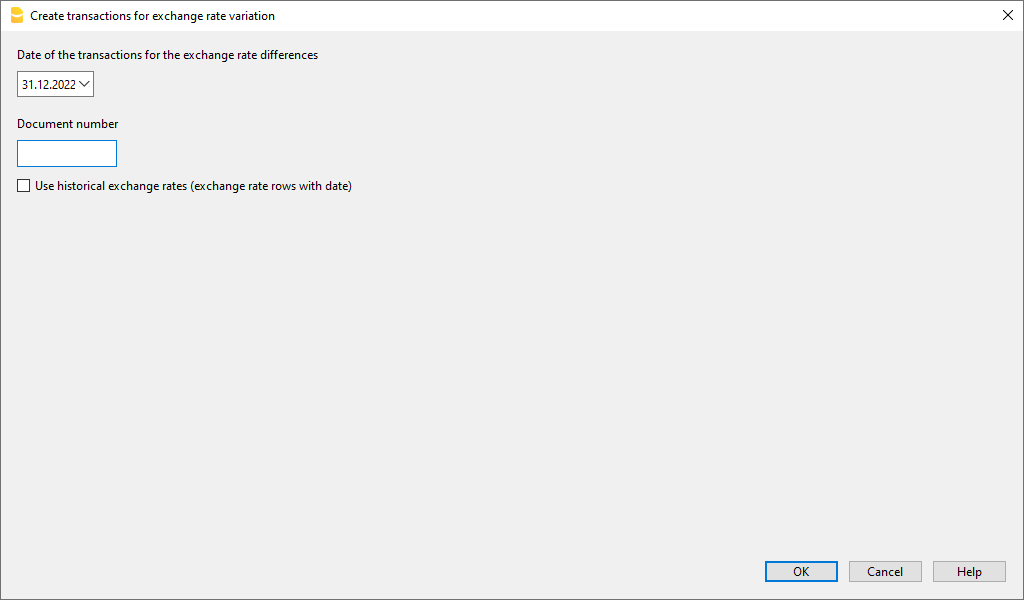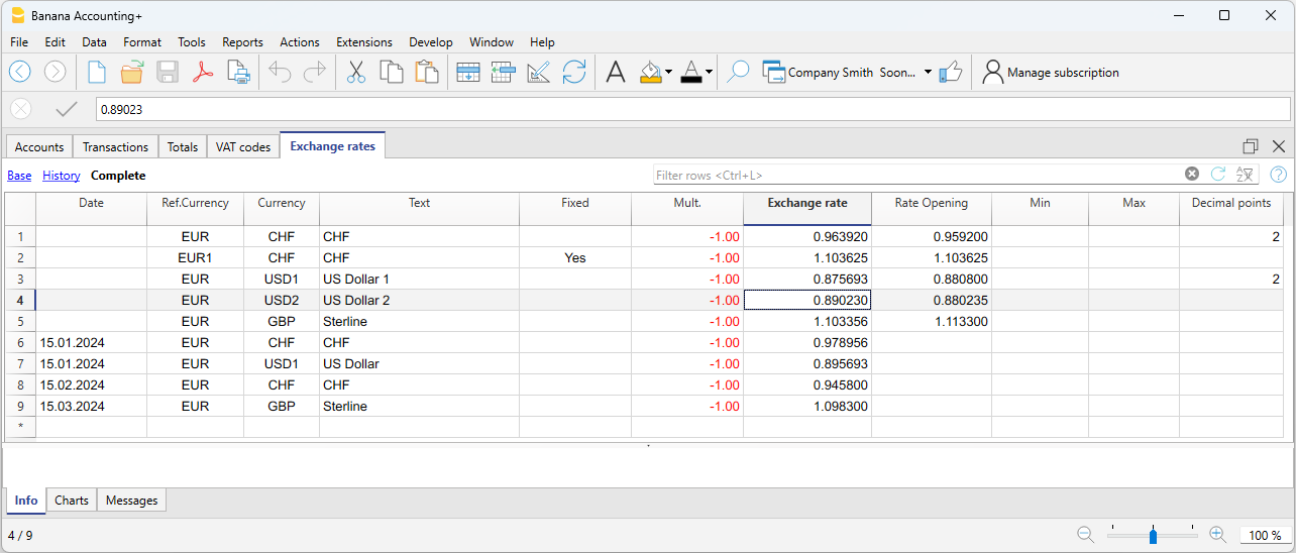# Exchange rate differences

Documentation •

## Create transactions for exchange rate differences

For theoretical aspects please visit the Revaluations and exchange rate differences page.

• The exchange differences transactions are adjustment transactions that balance out the foreign currency account balance with the basic currency calculated balance. In essence, it is a matter of re-adjusting the values ​​in the basic currency, taking into account exchange rate loss or gain, due to the fluctuations of the exchange rates.
• If these exchange rate differences are not recorded, there may be differences in the opening balances of the following year.
• The exchange rate differences can be calculated at the end of the closing year or during the accounting period (for example at the end of a quarter). In this case the historical changes can be useful, as they allow you to have different exchange rates at specific dates.
• The program calculates the exchange rate difference based on the balances at the specified date. It is therefore possible to calculate the exchange rate difference at a specific date, even if you have entered transactions after that date.
• For further explanations, see also the Exchange rate differences not booked page
• Exchange rate differences are not calculated on the accounts belonging to the profit and loss account. Exchange rate profit/losses, which are generated by exchange rate differences, are financial and non-operating items and are therefore not included in the determination of operating income or the profit and loss account.

## The Calculate exchange rate differences dialog

The Create transaction for exchange rate variation... command, from the Actions menu, calculates the revaluations for the foreign currencies accounts.#### Date of the transactions for the exchange rate differences

The program is able to calculate the exchange rate difference on a date even if there are postings beyond the date.

• Enter the date for your exchange rate differences transactions.
• The program will suggest the final date of the current month, related to the last entered transaction.
• If there are transactions for exchange rate differences with the same date, the program asks whether they should be replaced. The program considers the transactions for the exchange rates differences as existing if they have the same date, doc, description, accounts and currency and when there is no amount in the account currency.

#### Document number

Enter the document number your exchange rate differences transactions should have.

#### Use historical exchange rates (exchange rate rows with date)

• Use when option is not activated
• If in your Exchange rate table there are no historical exchange rates (exchange rates with a date) this option is not activated.
• The program will use the exchange rate in your Exchange rate table of the rows without date
• if you are using historical exchange rates for the year closure, be careful that the exchange rate used should be the same as the current one.
• Use when option is activated
• The program will indicate the date of the exchange rate, found in the Exchange rates table, that will be used to calculate the exchange rate difference.
This is going to be the exchange rate with a date equal or prior to the indicated date.
• When calculating exchange rate differences at year end we suggest this option not to be activated
• When booking exchange rate difference transactions at year end, the historical exchange rate must be the same as the current exchange rate, otherwise you get an error message saying that the exchange rate differences have not been calculated (even though they have been).

## Values used to create the transactions

#### For more information, we refer to our page Multi-currency transactions. Amount of the transaction

• Transactions for exchange rate differences are being created only for the accounts in foreign currency which, at the specified date, have a different balance in basic currency compared to the calculated one.
• For the amount in basic currency, the difference between the account balance in basic currency and the account balance in foreign currency converted in basic currency is being used.

#### Account balance

For the calculation of the exchange rate differences, the balances in the account currency and in basic currency are being used, at the specified date.

#### Exchange rate profit and exchange rate loss accounts

As exchange rate profit and loss accounts are being used, in order of priority:

1. The indicated accounts entered in the specific column of the chart of accounts.
2. The exchange rate profit & loss accounts indicated in the File and Accounting properties.

#### Position of inserted rows

When using the command, while in the Transactions table, the rows are being inserted at the position of the cursor.
Otherwise, they will be inserted at the end or at the previous position in case they are replacing existing transactions.

## Before using the command

1. In the File and Accounting properties  of the File menu, Foreign Currency section, make sure that the Exchange rate profit and loss accounts are being indicated. It is equally possible to indicate the same account for both the exchange rate profits or losses.
2. Make sure that the accounts in foreign currency are being updated and that the balances in foreign currency of these accounts (for example bank accounts) correspond to the balance indicated by the bank.
3. Update the current exchange rates of the Exchange rate table.
You should indicate the closing exchange rates or those of a period's end in the rows without a date, in the Exchange Rate column (do not modify the opening exchange rate in the Rate Opening column). In order to calculate the Exchange rate differences, the program uses the exchange rates of the rows without a date. If these last ones are absent, the program produces an error message.## Opening exchange rates for the New Year

To make the Opening balances of the New Year in Basic currency correspond exactly with the closing balances of the preceding year,  the Opening Exchange rates of the New Year, indicated in the Exchange Rate table, have to be the same as those being used for the closing of the accounting, so:

• The closing exchange rates have to be indicated in the Exchange rate column of the rows without a date;
• The opening exchange rates have to be indicated in the Rate Opening columns of the rows without a date.

The procedure of creating a new year or of the updating of the opening balances, copies the closing balances (Exchange rate column, rows without date) of the previous year into the opening exchange rates (Exchange rate table, Rate Opening column,  rows without date) of the new year's file.

## Exchange rate differences with cost centers

The Create transaction for exchange rate variation... command does not include any exchange rate differences present in cost centers in currencies other than the accounting currency. These differences must be recorded manually at the end of the year. The transaction should present only the accounting currency amount; indicate this amount first, then the cost center involved and the cost center currency. When the currency is entered, the amount in the currency of the accounting will be deleted and must be re-entered.

help_id